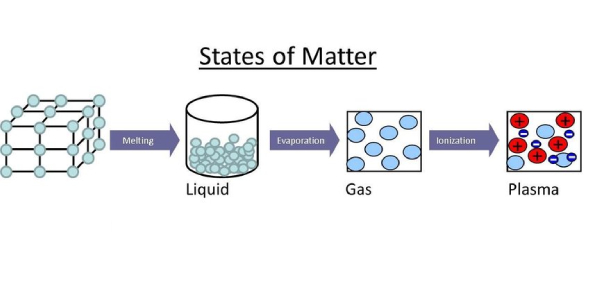# Test On States Of Matter! Chemistry Quiz

14 Questions | Total Attempts: 119Settings.

• 1.
Which of the following is not in the solid-state?
• A.

Water

• B.

Ice

• C.

Iron

• D.

Wood

• 2.
Which of the following is an amorphous solid?
• A.

Ice

• B.

Diamond

• C.

Rubber

• D.

Sand

• 3.
Which of the following states of matter has surface tension?
• A.

Solid

• B.

Liquid

• C.

Gas

• D.

Plasma

• 4.
Which one of the following states of matter is made of charged particles at high temperatures?
• A.

Solid

• B.

Liquid

• C.

Gas

• D.

Plasma

• 5.
Which of the following liquids has the greatest viscosity?
• A.

Water

• B.

Alcohol

• C.

Syrup

• D.

Mercury

• 6.
Some insects can walk on water due to its surface tension.
• A.

True

• B.

False

• 7.
The resistance to flowing is a measure of…
• A.

Surface tension

• B.

Temperature

• C.

Thermal energy

• D.

Viscosity

• 8.
A solid that has an orderly pattern of atoms is said to be ...
• A.

Crystalline

• B.

Amorphous

• C.

A liquid

• D.

Plasma

• 9.
Heat and thermal energy are the same except that ...
• A.

Heat is hotter

• B.

Heat flows

• C.

Thermal energy flows

• D.

Thermal energy is matter

• 10.
Which measurement is an average value for kinetic energy?
• A.

Thermal energy

• B.

Heat

• C.

Temperature

• D.

Viscosity

• 11.
Why does the sidewalk get hotter in summer than the grass?
• A.

Concrete has a low specific heat.

• B.

Grass has a low specific heat.

• C.

Grass has a higher viscosity.

• D.

Concrete contains water vapor.

• 12.
Which term refers to water becoming ice?
• A.

Condensation

• B.

Melting

• C.

Sublimation

• D.

Freezing

• 13.
Which term refers to the formation of dew on the grass?
• A.

Condensation

• B.

Sublimation

• C.

Vaporization

• D.

Precipitation

• 14.
Gaseous water that comes from boiling is steam. Gaseous water that is below the boiling point is called what?
• A.

Plasma

• B.

Vapor

• C.

Liquid

• D.

Amorphous

Related TopicsBack to top